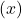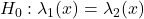# Conditional interval estimation for the ratio of shape parameters in power law process

Power law process (PLP) or Weibull process is used to model reliability growth
and is usually characterized by the intensity function. We investigate the
conditional confidence interval (CCI) for the ratio of two PLP shape parameters
following the rejection of the hypothesis. This interval
has been found to be possibly shorter than the unconditional confidence interval
(UCI). The conditional coverage probability (CCP) of the confidence interval is
obtained by computing the coverage probability under the conditional probability
density function. The CCP of the UCI is not uniformly greater than or less
than the nominal level. The UCI is appropriate only when one does not perform
any preliminary test (pre-test). However, if a pre-test is performed before the
construction of a confidence interval, then the appropriate interval is the CCI as
the pre-test affects subsequent inference procedures. The method is illustrated
on a data set.

Fulltext